Next: 7.5 Discussion Up: 7. Forward model for Previous: 7.3.5 Mental transformation

# 7.4 Data outside the training domain

This section explains why a multi-layer perceptron that is trained to map data points within a sensory manifold, may map data points outside its training domain closer to the manifold (section 7.3.2, figure 7.11, left). This phenomenon depends on the structure of the training domain. It is not a general property of MLPs.

First, I show that all image vectors have about the same length, independent of the position of the robot. Second, I give a two-dimensional synthetic example having the same property. Third, I explain theoretically why in the example data points outside the training domain are mapped closer to the domain. Last, I show that the abstract RNN does not have this property in the example.

We estimate the length of an image vector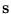(the sensory representation). Although the world-to-camera mapping was non-linear, the image of the obstacle circle was still close to circular (figure 7.3). Its area was further almost independent of the robot's position. Thus, we assume that also on the camera image, the obstacles form a circle with fixed area. Within this region, the robot can stay at any point. To obtain the sensory representation, the circle is subdivided into ten sectors centered at the robot's position (figure 7.15).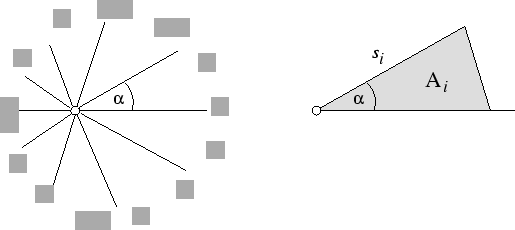Let si be the length of each sector, and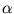be the angle of every sector (figure 7.15). Ifis small enough then the area of a sector is well approximated by

 Ai =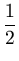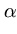si2 . (7.2)

Therefore, the squared length of an image vectorequals

 ||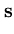||2 =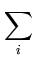si2 =Ai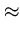Ao . (7.3)

Ao is the circle area enclosed by the obstacles. Ao is independent of the position of the robot. Therefore, all training patterns lie on a 10-sphere (embedded in ten dimensions) with radius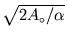.

In the synthetic example discussed in the following, a circle is mapped onto a circle; that is, input and output are two-dimensional, and the training domain is a circle in the input and in the output space. The two circles would coincide if input and output coordinate system were put on top of each other. Each point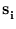in the input circle has in the second circle a target point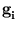that is rotated relative toby 23o around the origin. 200 training points uniformly distributed around the circle were generated. An MLP learned the mapping fromtofor all i = 1,..., 200. The MLP had a three layer structure composed of two input neurons, h = 5 hidden neurons, and two output neurons. In the hidden layer, the activation function was sigmoidal (tanh), and in the other layers, it was the identity function. Initially, the weights were drawn uniformly from the interval [-0.1; 0.1]. Using back-propagation in on-line mode, the network trained until convergence.

Figure 7.16 shows the result after training. Points outside the training domain (distance to the origin: 2.0) were mapped closer to the origin in the output space (distance around 1.5), and points inside the training domain (distance: 0.66) were mapped closer the unit circle (distance around 0.75).

Figure 7.16: Circle-to-circle mapping with 23o rotation. Input space (left) and output space (right) are shown. Training data are on a circle with radius 1. Square markers show test input (left) and corresponding output (right).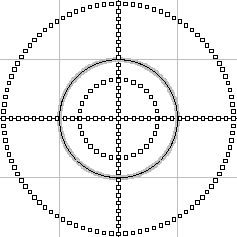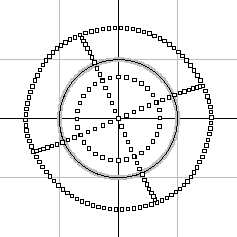In the following, this finding is studied theoretically. The MLP maps an inputto an output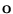,

 oi =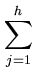vijtanh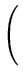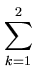ujksk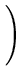, (7.4)

with h hidden units and weight matrices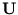and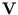. If the activation function in the hidden layer would be the identity function then the output scales as the input. Multiplying the input by a scalar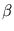gives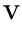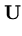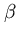=. (7.5)

Here, outliers are not mapped closer to the circle. Thus, the observed contraction is caused by the sigmoidal activation function.

In the example with the two-dimensional circle, it was observed that in the trained network, the column vectors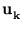ofwere approximately orthogonal and had unit length; the same held for the row vectors7.2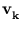of. Thus, we assume that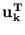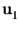=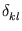and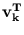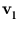=. With this assumption, it can be shown (appendix C.4) that pointsoutside the circle are mapped closer to the circle,

 |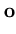| <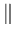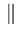. (7.6)

The theoretical explanation can be also extended to arbitrary dimensions with a hyper-sphere instead of a circle. In our robot task, however, the training patterns cannot cover all of the hyper-sphere because they are restricted to a two-dimensional manifold; in the synthetic example the whole circle is covered. This weakens the comparison.

The assumption=further predicts that the contraction effect decreases with increasing number of neurons h in the hidden layer. The assumption infers that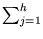u2jk = 1. Thus, the expectation value of u2jk equals 1/h. The argument of tanh is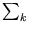ujksk. Here, the only random variables are {ujk}, since the statement should hold for all. Further, we assume that the expectation value of ujk is zero. Then, for all inputswith length, the expectation value of the squared tanh-argument can be written as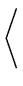ujksk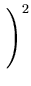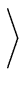=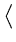u2jk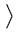sk2 =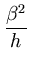. (7.7)

The absolute mean value of the tanh-argument decreases with increasing h. Therefore, the tanh-function gets closer to the identity function, and the contraction effect weakens.

This finding was tested with the above experiment for different values of h. The result is shown in table 7.4. The values were averaged over three separately trained networks and on 360 trials each. The length of input vectors was set to 2.0. This experiment is in agreement with the above theoretical prediction.

Table 7.4: Dependence of the mean contraction c =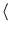||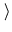/ |||| on the number of hidden neurons.
 hidden neurons c 5 0.78 10 0.85 15 0.89 20 0.91 25 0.92

Different from the MLP, the abstract RNN maintains the scale in the circle task (figure 7.17). The 200 pairs of circle points (,) were approximated using a mixture of five units, each with two principal components (using for training MPPCA-ext). The centers of the ellipsoids turned out to be evenly distributed around the circle. Figure 7.17 shows that the distance to the origin is consistent between input and output pairs. As in (7.5), the local linear mappings do not change the length of input patterns.

Figure 7.17: Circle-to-circle mapping with 23o rotation, using the abstract RNN. Input space (left) and output space (right) are shown. Training data are on a circle with radius 1. Square markers show test input (left) and corresponding output (right).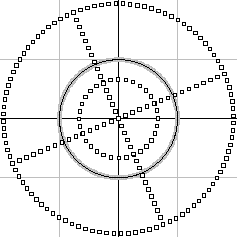Next: 7.5 Discussion Up: 7. Forward model for Previous: 7.3.5 Mental transformation
Heiko Hoffmann
2005-03-22